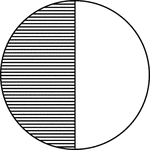### Circle, 1/2 Fraction

Illustration of a circle divided in half.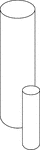### 2 Similar Cylinders

Illustration of 2 similar cylinders. The height and diameter of the smaller cylinder is half that of…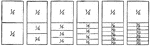### Fraction Comparisons

Rectangles comparing fraction values to one half: 1/4, 1/6, 1/8, 1/10, and 1/12.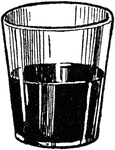### Half Full Glass

An illustration of a glass half full.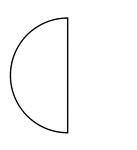### Half of a Fraction Pie

One half of a circle (vertical left).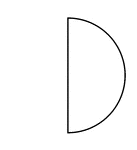### Half of a Fraction Pie

One half of a circle (vertical right).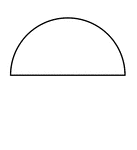### Half of a Fraction Pie

One half of a circle (horizontal top).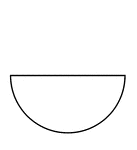### Half of a Fraction Pie

One half of a circle (horizontal bottom).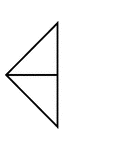### Fractions of 4-sided Polygon

2/4 of a 4 sided polygon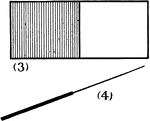### Rectangle, 1/2 Fraction

Illustration of a rectangle divided in half.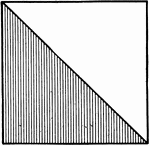### Square, 1/2 Fraction

Illustration of a square divided in half.